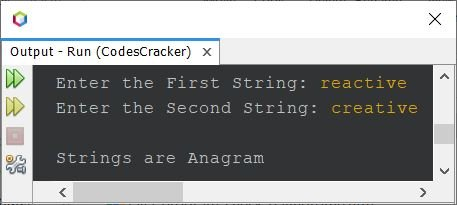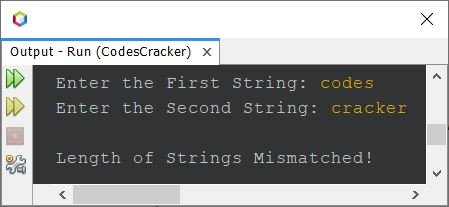# Java Program to Check Anagram or Not

Two strings are anagram, if one string can be re-arranged to form the other. For example, listen and silent are anagram strings. Because listen can be re-arranged to form silent and vice-versa.

Now the question is, write a Java program to check whether strings are anagram or not. The program given below is its answer:

```import java.util.Scanner;

public class CodesCracker
{
public static void main(String[] args)
{
String strOne, strTwo;
int lenOne, lenTwo, i, j, found=0, not_found=0;
Scanner scan = new Scanner(System.in);

System.out.print("Enter the First String: ");
strOne = scan.nextLine();
System.out.print("Enter the Second String: ");
strTwo = scan.nextLine();

lenOne = strOne.length();
lenTwo = strTwo.length();

if(lenOne == lenTwo)
{
for(i=0; i<lenOne; i++)
{
found = 0;
for(j=0; j<lenOne; j++)
{
if(strOne.charAt(i) == strTwo.charAt(j))
{
found = 1;
break;
}
}
if(found == 0)
{
not_found = 1;
break;
}
}
if(not_found == 1)
System.out.println("\nStrings are not Anagram");
else
System.out.println("\nStrings are Anagram");
}

else
System.out.println("\nLength of Strings Mismatched!");
}
}```

Here is its sample run with user input reactive as first and creative as second string:Here is another sample run with user input codes and cracker as two strings:In above program, I've checked the length of both the string first. If the length will be equal, then further proceed, otherwise print the message for unequal length.

Now compared the first character of the first string to all the character of second string, one by one. Then compared the second character of the first string to all the character of second string, one by one, and so on.

While comparing, if any character of the first string gets matched to the character of the second string, then 1 gets initialized to the found, and exists from the loop using break. And if there is no match found, then 1 will not get initialized to the found. Therefore after exiting the inner loop, I've checked and printed the message, whether the strings are anagram or not.

#### Same Program in Other Languages

Java Online Test

« Previous Program Next Program »